cancel
Showing results for
Did you mean:

Fabric is Generally Available. Browse Fabric Presentations. Work towards your Fabric certification with the Cloud Skills Challenge.Helper I

## Analyzing Sales Data line items

Hi there,

I have sales data line items for the last 3 years that looks like the below example, and I would need some help with analyzing this data:

 ____Period____| ____Product____| ____Customer____| ____Volume____| Apr 2019 Product 1 Customer A 100 May 2019 Product 1 Customer A 150 Jun 2019 Product 1 Customer A 170 Jul 2019 Product 1 Customer A 160 Aug 2019 Product 1 Customer A 170 Apr 2020 Product 1 Customer A 110 May 2020 Product 1 Customer A 160 Jun 2020 Product 1 Customer A 180 Jul 2020 Product 1 Customer A 170

My questions are the following:

1. The company has a custom calendar, e.g Period 1 is July, Period 2 is August, etc. How can I calculate the year-to-date volume this year and compare it with the year-to-date volume last year? Also, this year-to-date calculation need to be updated based on which month is the most recent full month data (e.g in November, year-to-date would mean the period between July and October)
2. I need to calculate rolling trends based on a selection in a slicer: e.g if the user selects July, then I need to summarize the rolling 3 months this year (May, June, July) and compare the growth with the rolling 3 months last year. But if the user updates the slicer to February then the calculation also refreshes to capture the last three months (December, January and February).

Thanks,

kukszi

1 ACCEPTED SOLUTIONSuper User

Hi,

Hope this helps.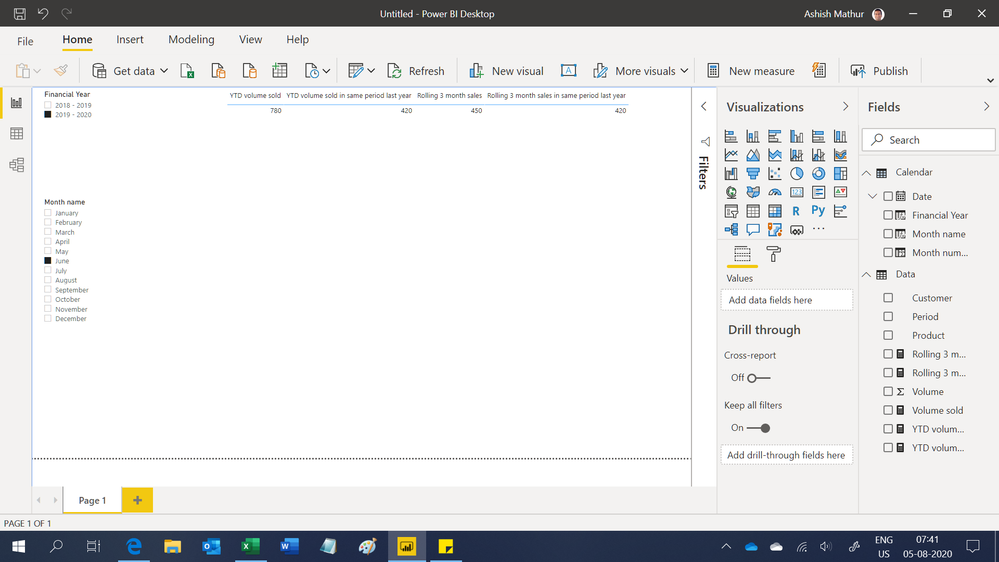Regards,
Ashish Mathur
http://www.ashishmathur.com
4 REPLIES 4Community Support

Hi @kukszi

I build a table like yours to have a test.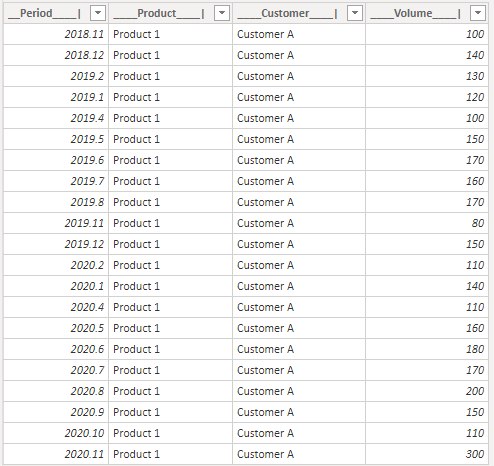I build measure to achieve your goals.

1.
``````Year-to-date volume this year =

VAR _Selected = SELECTEDVALUE(Calender[Date].[MonthNo])

VAR _MAXMon =

MAXX (

FILTER ( ALL ( 'Table' ), 'Table'[__Period____|].[Year] = YEAR ( TODAY () ) ),

'Table'[__Period____|].[MonthNo]

)

RETURN

CALCULATE (

SUM ( 'Table'[____Volume____|] ),

FILTER (

ALL ( 'Table' ),

'Table'[__Period____|].[Year] = YEAR ( TODAY () )

&& 'Table'[__Period____|].[MonthNo] < _MAXMon

&& 'Table'[__Period____|].[MonthNo] >= _Selected

)

)``````
``````Year-to-date volume last year =

VAR _Selected = SELECTEDVALUE(Calender[Date].[MonthNo])

VAR _MAXMon =

MAXX (

FILTER ( ALL ( 'Table' ), 'Table'[__Period____|].[Year] = YEAR ( TODAY () ) ),

'Table'[__Period____|].[MonthNo]

)

RETURN

CALCULATE (

SUM ( 'Table'[____Volume____|] ),

FILTER (

ALL ( 'Table' ),

'Table'[__Period____|].[Year]

= YEAR ( TODAY () ) - 1

&& 'Table'[__Period____|].[MonthNo] < _MAXMon

&& 'Table'[__Period____|].[MonthNo] >= _Selected

)

)``````

When My latest value is in Nov 2020,Result:

Default: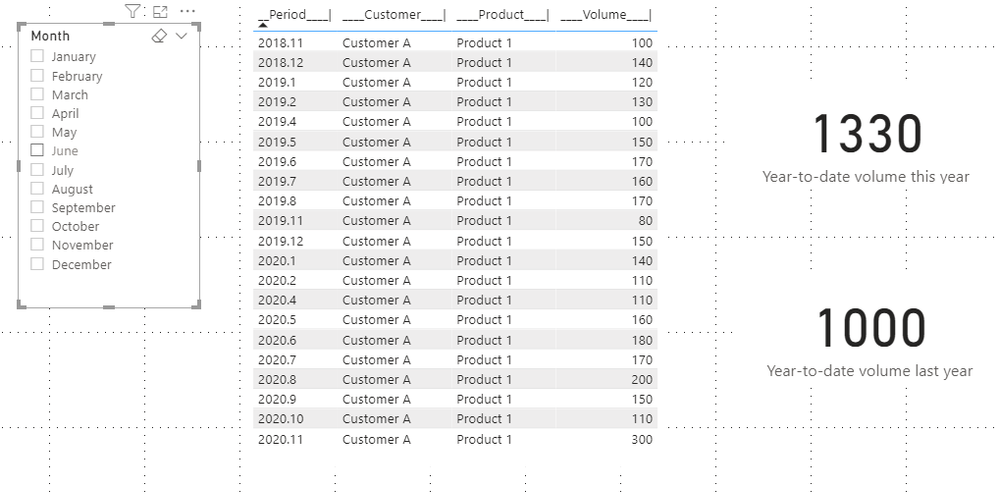Select July:2.

``````Rolling 3 months last year =

VAR _selectedmonth =

SELECTEDVALUE ( Calender[Date].[MonthNo] )

VAR _a =

CALCULATE (

SUM ( 'Table'[____Volume____|] ),

FILTER (

ALL ( 'Table' ),

'Table'[__Period____|].[Year]

= YEAR ( TODAY () ) - 1

&& 'Table'[__Period____|].[MonthNo] <= _selectedmonth

&& 'Table'[__Period____|].[MonthNo] >= _selectedmonth - 2

)

)

VAR _b =

CALCULATE (

SUM ( 'Table'[____Volume____|] ),

FILTER (

ALL ( 'Table' ),

'Table'[__Period____|].[Year]

= YEAR ( TODAY () ) - 2

&& 'Table'[__Period____|].[MonthNo] <= 12

&& 'Table'[__Period____|].[MonthNo] > 12 - 3 + _selectedmonth

)

)

RETURN

IF ( _selectedmonth <= 2, _a + _b, _a )``````
``````Rolling 3 months this year =

VAR _selectedmonth =

SELECTEDVALUE ( Calender[Date].[MonthNo] )

VAR _a =

CALCULATE (

SUM ( 'Table'[____Volume____|] ),

FILTER (

ALL ( 'Table' ),

'Table'[__Period____|].[Year] = YEAR ( TODAY () )

&& 'Table'[__Period____|].[MonthNo] <= _selectedmonth

&& 'Table'[__Period____|].[MonthNo] >= _selectedmonth - 2

)

)

VAR _b =

CALCULATE (

SUM ( 'Table'[____Volume____|] ),

FILTER (

ALL ( 'Table' ),

'Table'[__Period____|].[Year]

= YEAR ( TODAY () ) - 1

&& 'Table'[__Period____|].[MonthNo] <= 12

&& 'Table'[__Period____|].[MonthNo] > 12 - 3 + _selectedmonth

)

)

RETURN

IF ( _selectedmonth <= 2, _a + _b, _a )``````

Result:

July: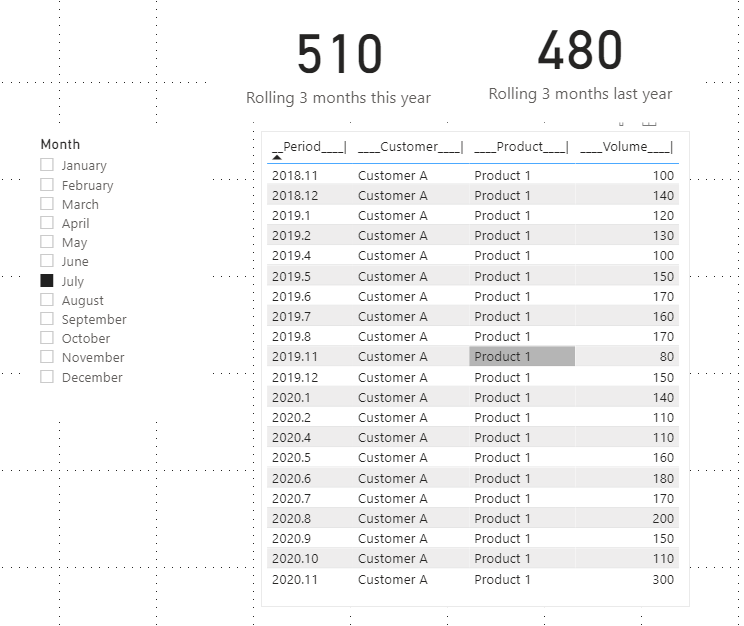February: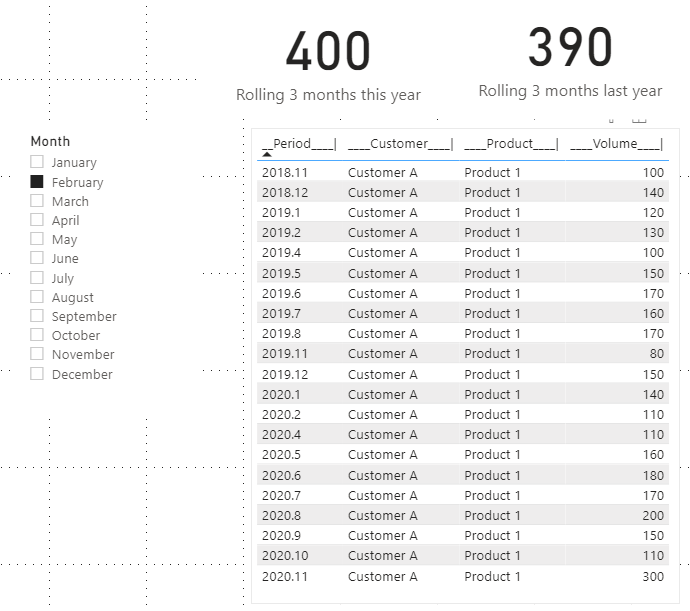Best Regards,

Rico Zhou

If this post helps, then please consider Accept it as the solution to help the other members find it more quickly.Super User

@kukszi , find a calendar of your choice -

https://community.powerbi.com/t5/Community-Blog/Creating-Financial-Calendar-Decoding-Date-and-Calend...

YTD can take year-end date. Your QTR is will work with time intelligence

``````Last QTD Sales = CALCULATE(SUM(Sales[Sales Amount]),DATESQTD(dateadd('Date'[Date],-1,QUARTER)))
Last complete QTD Sales = CALCULATE(SUM(Sales[Sales Amount]),DATESQTD( ENDOFQUARTER(dateadd('Date'[Date],-1,QUARTER))))
``````

``````YTD Sales = CALCULATE(SUM(Sales[Sales Amount]),DATESYTD('Date'[Date],"12/31"))
Last YTD Sales = CALCULATE(SUM(Sales[Sales Amount]),DATESYTD(dateadd('Date'[Date],-1,Year),"12/31"))
This year Sales = CALCULATE(SUM(Sales[Sales Amount]),DATESYTD(ENDOFYEAR('Date'[Date]),"12/31"))
Last year Sales = CALCULATE(SUM(Sales[Sales Amount]),DATESYTD(ENDOFYEAR(dateadd('Date'[Date],-1,Year)),"12/31"))
Last to last YTD Sales = CALCULATE(SUM(Sales[Sales Amount]),DATESYTD(dateadd('Date'[Date],-2,Year),"12/31"))
Year behind Sales = CALCULATE(SUM(Sales[Sales Amount]),dateadd('Date'[Date],-1,Year))
``````

rolling

``````Rolling 12 = CALCULATE(sum(Sales[Sales Amount]),DATESINPERIOD('Date'[Date],ENDOFMONTH(Sales[Sales Date]),-12,MONTH))
Rolling 12 = CALCULATE(sum(Sales[Sales Amount]),DATESINPERIOD('Date'[Date ],MAX(Sales[Sales Date]),-12,MONTH))
Rolling 3= CALCULATE(sum(Sales[Sales Amount]),DATESINPERIOD('Date'[Date],staroffmonth(Sales[Sales Date]),3,MONTH))
Rolling 3 till last month = CALCULATE(sum(Sales[Sales Amount]),DATESINPERIOD('Date'[Date],ENDOFMONTH(dateadd(Sales[Sales Date],-1,month)),-3,MONTH))
Rolling 3 till last 2 month = CALCULATE(sum(Sales[Sales Amount]),DATESINPERIOD('Date'[Date],ENDOFMONTH(dateadd(Sales[Sales Date],-2,month)),-3,MONTH))
Rolling 3 till last 1 month = CALCULATE(sum(Sales[Sales Amount]),DATESINPERIOD('Date'[Date],ENDOFMONTH(dateadd(Sales[Sales Date],-1,month)),-3,MONTH))
Rolling 12 till last month = CALCULATE(sum(Sales[Sales Amount]),DATESINPERIOD('Date'[Date],ENDOFMONTH(dateadd(Sales[Sales Date],-1,month)),-13,MONTH))
Rolling 3 till last 3 month = CALCULATE(sum(Sales[Sales Amount]),DATESINPERIOD('Date'[Date],ENDOFMONTH(dateadd(Sales[Sales Date],-3,month)),-3,MONTH))
``````

refer for more deatils

Power BI — YTD Questions — Time Intelligence 1–5
https://medium.com/@amitchandak.1978/power-bi-ytd-questions-time-intelligence-1-5-e3174b39f38a
Power BI — QTD Questions — Time Intelligence 2–5
https://medium.com/@amitchandak.1978/power-bi-qtd-questions-time-intelligence-2-5-d842063da839
Power BI — MTD Questions — Time Intelligence 3–5
https://medium.com/@amitchandak.1978/power-bi-mtd-questions-time-intelligence-3-5-64b0b4a4090e

To get the best of the time intelligence function. Make sure you have a date calendar and it has been marked as the date in model view. Also, join it with the date column of your fact/s. Refer :
https://www.archerpoint.com/blog/Posts/creating-date-table-power-bi
https://www.sqlbi.com/articles/creating-a-simple-date-table-in-dax/

See if my webinar on Time Intelligence can help: https://community.powerbi.com/t5/Webinars-and-Video-Gallery/PowerBI-Time-Intelligence-Calendar-WTD-Y...Super User

Hi,

Hope this helps.Regards,
Ashish Mathur
http://www.ashishmathur.comSuper User

Hey @kukszi ,

https://www.daxpatterns.com/time-patterns/

Regards,

Tom

Did I answer your question? Mark my post as a solution, this will help others!

Proud to be a Super User!
I accept Kudos 😉
Hamburg, GermanyAnnouncements#### Power BI Monthly Update - November 2023

Check out the November 2023 Power BI update to learn about new features.#### Fabric Community News unified experience

Read the latest Fabric Community announcements, including updates on Power BI, Synapse, Data Factory and Data Activator.#### Exclusive opportunity for Women!

Join us for a free, hands-on Microsoft workshop led by women trainers for women where you will learn how to build a Dashboard in a Day!#### The largest Power BI and Fabric virtual conference

130+ sessions, 130+ speakers, Product managers, MVPs, and experts. All about Power BI and Fabric. Attend online or watch the recordings.Top Solution Authors
Top Kudoed Authors
Users online (2,352)